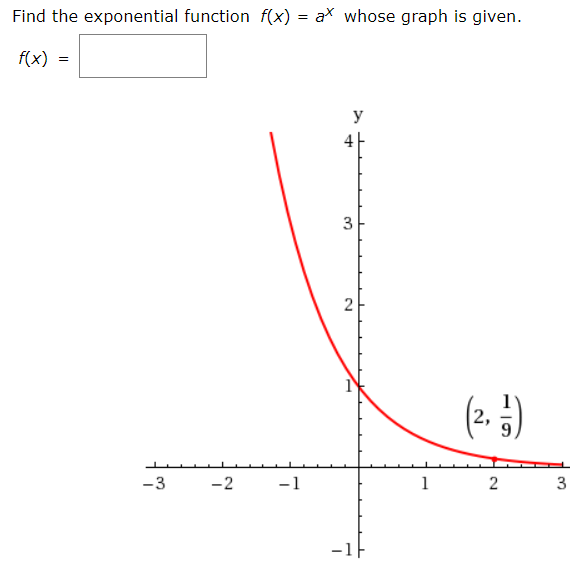# ax whose graph is given.Find the exponential function f(x)f(x)y2,-3-2-1-13.2.2.

Question
12 viewshelp_outlineImage Transcriptioncloseax whose graph is given. Find the exponential function f(x) f(x) y 2, -3 -2 -1 -1 3. 2. 2. fullscreen
check_circle

Step 1

Given function is f(x) = ax whose graph is given below

Step 2

Since, (2, 1/9) satisfy the eq...

### Want to see the full answer?

See Solution

#### Want to see this answer and more?

Solutions are written by subject experts who are available 24/7. Questions are typically answered within 1 hour.*

See Solution
*Response times may vary by subject and question.
Tagged in

### Functions